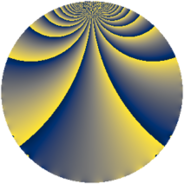# Properties

 Label 2280.2.fLevel $2280$ Weight $2$ Character orbit 2280.f Rep. character $\chi_{2280}(229,\cdot)$ Character field $\Q$ Dimension $216$ Sturm bound $960$

# Related objects

## Defining parameters

 Level: $$N$$ $$=$$ $$2280 = 2^{3} \cdot 3 \cdot 5 \cdot 19$$ Weight: $$k$$ $$=$$ $$2$$ Character orbit: $$[\chi]$$ $$=$$ 2280.f (of order $$2$$ and degree $$1$$) Character conductor: $$\operatorname{cond}(\chi)$$ $$=$$ $$40$$ Character field: $$\Q$$ Sturm bound: $$960$$

## Dimensions

The following table gives the dimensions of various subspaces of $$M_{2}(2280, [\chi])$$.

Total New Old
Modular forms 488 216 272
Cusp forms 472 216 256
Eisenstein series 16 0 16

## Trace form

 $$216q + 216q^{9} + O(q^{10})$$ $$216q + 216q^{9} + 8q^{10} + 16q^{14} - 8q^{25} + 16q^{26} - 24q^{30} - 48q^{34} + 32q^{39} + 4q^{40} + 16q^{41} - 32q^{44} + 8q^{46} - 216q^{49} + 36q^{50} + 32q^{55} - 104q^{56} + 20q^{60} + 48q^{64} + 48q^{65} - 16q^{66} - 76q^{70} - 64q^{71} - 40q^{74} + 80q^{80} + 216q^{81} + 32q^{84} + 32q^{86} + 80q^{89} + 8q^{90} + 16q^{94} + 40q^{96} + O(q^{100})$$

## Decomposition of $$S_{2}^{\mathrm{new}}(2280, [\chi])$$ into newform subspaces

The newforms in this space have not yet been added to the LMFDB.

## Decomposition of $$S_{2}^{\mathrm{old}}(2280, [\chi])$$ into lower level spaces

$$S_{2}^{\mathrm{old}}(2280, [\chi]) \cong$$ $$S_{2}^{\mathrm{new}}(40, [\chi])$$$$^{\oplus 4}$$$$\oplus$$$$S_{2}^{\mathrm{new}}(120, [\chi])$$$$^{\oplus 2}$$$$\oplus$$$$S_{2}^{\mathrm{new}}(760, [\chi])$$$$^{\oplus 2}$$